# Parameter Tuning of Brushless DC Motor for Improving Control Effect with Worm Algorithm

Parameter Tuning of Brushless DC Motor for Improving Control Effect with Worm Algorithm
College of Mechanical and Control Engineering, Guilin University of Technology, Guilin 541000, Guangxi, China

Corresponding Author Email:
qinjh2@sina.com
Page:
229-235
|
DOI:
https://doi.org/10.18280/ejee.230307
5 March 2021
|
Accepted:
8 April 2021
|
Published:
21 June 2021
| Citation

OPEN ACCESS

Abstract:

Aiming at the problem of low control precision and small applicable scope caused by adjusting control parameters in Ziegler-Nichols (ZN) method, a parameter tuning method based on Worm algorithm (WOA) is proposed for Brushless DC motor. Firstly, the model of speed control is established by proportional integral method for Brushless DC motor with two - phase conduction and three - phase full bridge drive. Then the fitness function of the controller is constructed by the Integral Absolute Error (IAE). Finally, the early optimization process, the later movement rule and the peak extraction rule are determined for WOA, and the controller parameter tuning process is designed. Simulation results under constant and sinusoidal conditions show the effectiveness of the proposed method. WOA was compared with ZN, genetic algorithm (GA), differential evolution algorithm (DE) and particle swarm optimization algorithm (PSO) in the experiment. The experimental results show that the control effect (CE) of WOA under uniform speed has been improved by 2.56% on average, and has been improved by 16.93% on average under sinusoidal speed. Compared with previous methods, this method can be used for parameter adjustment of complex control with higher control precision.

Keywords:

BLDCM, parameter tuning, WOA, speed control

1. Introduction

The speed is one of the important control objects for the Brushless DC Motor (BLDCM). Due to the real-time and the coupling of the BLDCM, the traditional method with Ziegler-Nichols (ZN) could not satisfy the operational requirements in the practical applications such as the cooler [1, 2] and the electric vehicle . BLDCM not only needs more complex control, but also requires higher control precision in the control of robot. In order to reduce the adjustment difficulty and to improve the control accuracy under complex control requirements, the experts use the intelligent algorithm to adjust the control parameters.

Cao  designed an optimization method for the direct drive servo system based on the genetic algorithm, and the method verified that it has the good dynamic response characteristics for the permanent magnet synchronous motor. Ma et al.  designed a control strategy of the controller based on the fuzzy neural network. By using the strategy, the device can prevent the danger of mine hoist from decelerating at low cost, and the safety and reliability of mine hoist are greatly improved. El-Wakeel et al.  proposed a technology to determine the parameters of the speed-controller for the permanent magnet brushless DC motor based on the hybrid particle swarm optimization and the bacterial foraging. It is concluded that the technology is more effective in improving the step response characteristics and achieving the expected performance index. Zhou et al.  proposed the improved spotted hyena optimization algorithm (ISHO) with nonlinear convergence factor to optimize the proportional integral derivative (PID) parameter for automatic voltage regulator (AVR). The advantages of the proposed algorithm are verified by experiments in terms of solution accuracy and convergence speed. Jing proposed a genetic fuzzy immune PID algorithm to achieve a constant speed in this study . The results showed that the algorithm has a significant effect. Cui et al.  proposed an improved monarch butterfly algorithm based on local search and differential evolution. The experiment results showed that the superiority of the proposed algorithm in PID tuning]. Yu et al.  proposed an improved PSO algorithm that optimized the control PID parameters of a specific robot. The experimental results verify the effectiveness of the proposed method.

In addition, parameter tuning methods based on other intelligent algorithms are referred to this paper. For example, genetic algorithm (GA) [11-13], fuzzy control algorithm , particle swarm optimization algorithm (PSO) , differential evolution algorithm (DE) , and fuzzy neural network .

The methods of the parameters adjusted based on the above intelligent algorithm can control the object effectively. However, the intelligent algorithms need lots of calculations to meet the requirements of control accuracy. Therefore, it is especially important to find an intelligent algorithm with fewer calculations and higher accuracy. The advantages of the few input parameters, the stable calculation result, and the fast convergence speed of the worm algorithm are determined by the unique structure and calculation method. The purpose to reduce the total deviation of speed control and improving the operating effect for BLDCM is met by establishing the speed control model, determining the fitness function standard of controller, and designing the tuning method of controller parameters based on the worm algorithm (WOA) .

2. BLDCM Speed Control Model

The premise of the experimental is to establish the model. The model of the BLDCM with the two-phase conduction is universal and representative, which is shown in Figure 1.

## 1.png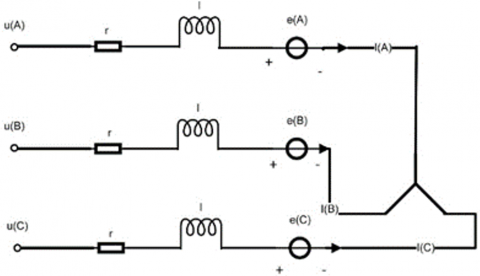Figure 1. Equivalent circuit of brushless DC motor

As shown in Figure 1, the BLDCM adopts Y-type connection mode, and there is two-phase conduction in each state during the operation. The voltage balance equation is obtained without considering the influence of the eddy current effect and the hysteresis while the excitation current and the motor compensation are supposed in an ideal state.

$\left[\begin{array}{l}u_{A} \\ u_{B} \\ u_{C}\end{array}\right]=\left[\begin{array}{lll}r & 0 & 0 \\ 0 & r & 0 \\ 0 & 0 & r\end{array}\right]\left[\begin{array}{l}I_{A} \\ I_{B} \\ I_{C}\end{array}\right]+\left[\begin{array}{lll}l & 0 & 0 \\ 0 & l & 0 \\ 0 & 0 & l\end{array}\right] \frac{d}{d t}\left[\begin{array}{c}I_{A} \\ I_{B} \\ I_{C}\end{array}\right]+\left[\begin{array}{c}e_{A} \\ e_{B} \\ e_{C}\end{array}\right]$                       (1)

In the formular, uA, uB and uC are the phase voltage of stator winding (V); r is the resistance of each phase (Ω); IA, IBand IC are the stator phase winding current (A); d is the differential operator; eA, eB, and eC are the reverse electromotive force of the stator phase winding (V); l is the self-inductance of the winding (H).

Eq. (1) can be simplified as:

$u_{A}(t)=r_{A} I_{A}(t)+l_{A} \frac{d I_{A}(t)}{d t}+e(t)$                     (2)

In the formular, uA is the armature voltage (V), e is the reverse potential (V), IA is the armature current (A), rA is the winding resistance (Ω), lA is the armature inductance (H).

The relationship between the reverse potential and the rotational speed is shown in Eq. (3).

$e(t)=k_{e} n(t)$                 (3)

In the formula, ke is the back electromotive force $(\mathrm{V} \cdot \min / r)$the speed of BLDCM $(r / \min )$ he electromagnetic torque equation (Te) of the BLDCM is shown in Eq. (4).

$T_{e}(t)=\frac{e_{A} I_{A}+e_{B} I_{B}+e_{C} I_{C}}{n}=k_{t} i_{d}(t)$                         (4)

In the formula, kt is the torque constants $(N . m / A)$, Te is the electromagnetic torque $(N . m)$.

The mechanical motion equation is as follows:

$J \frac{d n_{t}}{d t}=T_{e}(t)-b_{v}$              (5)

In the formula, J is the moment of inertia (kg·m2), bv is viscosity coefficient (N.m.s).

Open loop transfer function of BLDCM transformed by Laplace method:

$G(s) H(s)=\frac{k_{t} k_{e}}{l_{a} J s^{2}+\left(r_{a} J+l_{a} b_{n}\right) s+r_{a} b_{v}}$                        (6)

In the formula: ke is the back electromotive force constant $(\mathrm{V} \cdot \min / \mathrm{r})$. kt is the motor torque constant $(N . m / A)$, J is the moment of inertia (kg·m2), bv is the viscosity coefficient (N.m.s), ra is the winding resistance of the BLDCM (Ω), la is the armature inductance (H).

When the load torque is 0, the open-loop function of BLDCM speed with PI control by Laplace method is obtained.

$G G(s) H(s)=\frac{k_{t} k_{e} K_{p}\left(T_{i} s+1\right)}{T_{i} s\left(l_{a} s+r_{a}\right)\left(J s+b_{v}\right)} =\frac{k_{t} k_{e} K_{p} K_{i}}{s\left(l_{\alpha} s+r_{a}\right)\left(J s+b_{n}\right)}$               (7)

In the formula, kp is the proportion gain coefficient, Ti is the integral time, and $K_{i}=K_{p} / T_{i}$ is the integral gain coefficient.

3. Method

The controller parameters with good control effect, high control accuracy, high reliability and strong identification are obtained by tuning the parameters of BLDCM.

3.1 WOA controller parameter optimization

The best control effect of the speed is the key of tuning the parameters of the controller for the BLDCM. Because of the short length in coding, the strong ability in searching and the stable direction in optimizing. The decimal coding is adopted:

$K=\left\{K_{p}, K_{i}\right\}$             (8)

In the formula, K is the optimal individual of the algorithm, and it is the overall control parameter of the controller also. kp and ki are the dimensions of the optimal individuals. kp is used to increase the control sensitivity, to improve the regulation speed and to reduce the steady-state error. ki is used to eliminate steady-state error and to improve control accuracy.

3.2 Determination of fitness function

There are many evaluation indexes for the control effect of the motor speed. The method proposed in this paper is to reduce the total deviation of the BLDCM during the operation. Therefore, the Integral of Absolute Error (IAE) [19, 20] is used as the quantitative standard of the effect for the speed control (fitness function).

$F I T=\int_{0}^{\infty}|e(t)| d t$                      (9)

In the formula: FIT is the quantitative value of the effect for the speed control; $\int_{0}^{\infty}|e(t)| d t$ is the sum of absolute deviation among the actual speed and the expected speed during the operation time. Obviously, the value of FIT representative the adaptability of evolutionary individual K in the WOA. The smaller FIT, the better adaptive ability of evolutionary individuals. It shows that the stronger the adaptive ability of the evolutionary individual, the better the control effect of the controller on the BLDCM.

3.3 Rules of design

The rules of WOA are composed by early optimization rules, later moving rules and peak extraction rules . The specific rules of WOA are designed as follows:

Early optimization rules. The early optimization can find all the peaks in the interval and lay the foundation for the later optimization. The convergence speed and the computational efficiency of the WOA are improved by comparing the control effect (fitness value) of individual current position with that of adjacent individual. The parameter of the movement is set to 0 when the control effect of central individual is better than that of adjacent individual, otherwise the parameter of the movement is set to 1. The early optimization rule satisfied as:

$K_{p}(l)=\left(K_{p}(M A X)-K_{p}(M I N)\right) *(l-1) /(m-1)^{(l=1,2, \cdots, m)}$

$K_{i}(j)=\left(K_{i}(M A X)-K_{i}(M I N)\right) *(j-1) /(n-1)^{(j=1,2, \cdots, n)}$

Fitness $(K(l, j))=F I T\left(K_{p}(l), K_{i}(j)\right)$

$\left\{\begin{array}{c}\text { Forward }(l, j)=0 \text { Fitness }(K(l, j)) \text { is } M A X \\ \text { Forward }(l, j)=1 \text { else }\end{array}\right.$                             (10)

In the formula, the proportional gain coefficient and the integral gain coefficient of the algorithm individual K are $K_{p}(l)$ and $K_{i}(j)$ when the position is (l, j); the maximum and minimum values of the proportional gain coefficient are $K_{p}(M A X)$ and $K_{p}(M I N) ; K_{i}(M A X)$ and $K_{i}(M I N)$ are the maximum and minimum values of the integral gain factor; $\mathrm{m}$ and $\mathrm{n}$ are the number of individual values in the dimensions of the proportional and the integral; the control effect of Fitness $(K(l, j))$ is as the individual $K(l,$, j) for the BLDCM (Fitness Value); Forward $(l, j)$ is the moving coefficient of the individual $K(l, j)$.

Later movement rules. The later movement is used to find the better parameters of the controller under the results of the early optimization. The excellent individuals in the early search process are moved in a certain way at the later stage. K(l, j) is treated as a central individual during the later movements. The rules of the movement rules are used for adjacent individuals K(l-1, j), K(l+1, j), K(l, j-1) and K(l, j+1) to approach a central individual. The movement rules are as follows.

$K_{p}^{\prime}(q)=K_{p}(q)+\frac{K_{p}(l)-K_{p}(q)}{2}$

$K_{i}^{\prime}(\omega)=K_{i}(\omega)+\frac{K_{i}(j)-K_{i}(\omega)}{2}$

$(q=l-1, l+1 ; \omega=j-1, j+1)$                             (11)

In the formula: $K_{p}^{\prime}(q)$ and $K_{i}^{\prime}(\omega)$ are the coordinates to be reached by the next convergence of the adjacent individuals; $K_{p}(q)$ and $K_{i}(\omega)$ are the current coordinates of adjacent individuals; the coordinates of $K_{p}(l)$ and $K_{i}(j)$ as the central individuals; q and ω means that the center coordinate point corresponds to the left or right in space, and the adjacent individuals up or down.

In order to ensure that the central individual of the subgroup is always the local optimal value, and the adjacent individuals always move to the local optimal value. The central individual is replaced by the adjacent individuals when the fitness value of the adjacent individuals better than central individual in the process of moving.

The extractive rules of the peak. Through the early optimization rules, the local peak area of the function is surrounded by the central individual and the adjacent individual. The central individual with high fitness is approached by the adjacent with low fitness when the central individual and the adjacent individual are on the same side of the same peak. The adjacent individuals move to the centre before the fitness exceeds the central individual when the central individual and the adjacent individual are on different sides of the same peak. According to the post move rule, the adjacent individuals are close to the centre individuals until they reach the peak. The optimization process is completed after G cycles, and the individual with the best fitness is the best controller.

3.4 Parameter setting process

The steps to tune the parameters of the speed controller based on the WOA is shown as Figure 2.

The number of the initial individuals are selected to m*n in the defined interval and their fitness values were calculated. According to the early optimization rules, the move parameter is set to 0 when the fitness value of the individual is higher than that of its neighbors, otherwise the move parameter is set to 1.

Individuals K(l, j) with a movement parameter of 0 form a subgroup with their neighbors as the central individuals. The subpopulation individuals move towards the centre through the later movement rules.

The central individual of the current subpopulation is replaced by the individual with the largest adaptation value to ensure that the adaptation of the central individual is always greater than that of its neighbors.

When the number of the iterations is the maximum number of the evolutionary generations (G), the individual with the maximum adaptation value is output as the optimal individual through the extractive rules of the peak, and the calculation is terminated.

## 2.pngFigure 2. Flow chart of worm algorithm

4. Simulations, Experiments, Result and Discussion

4.1 Simulations of BLDCM

The experiments were conducted by the Robomaster M2006 P36 series BLDCM (Figure 3). The torque constant kt was $1.4(\mathrm{~N} \cdot \mathrm{m} / \mathrm{A})$, the winding inductance $l_{a}$ was $64.22(\mu \mathrm{H})$, the moment of inertia of J was 0.0008 (kg·m2), the winding resistance $r_{a}$ was 461 (), the viscous coefficient of bv was 0.001 (N.m.s), back emf constant for ke was 32.96 $(r / V)$, the simulation time was 0.2 s, the population number was 20, the number of iterations was 20 and the evaluation standard used IAE.

## 3.jpg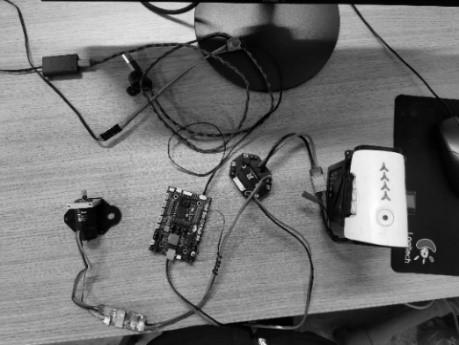Figure 3. Brushless DC motor

The performance of speed control was analyzed for the constant speed and the sinusoidal speed. In the simulation, the load torque was 3 N.m, and the load generation time was 0.1 s. The Constant speed was 300 rpm. The upper limit of the Sinusoidal Speed was 300 rpm, the lower limit was 100 rpm, and the frequency was 100 rad/s. In order to make the result clearer, the Relative Error curve $\left(R E_{\text {WoA-j }}(t)\right)$ of (a) - (d) and the Cumulative Error curve $\left(C E_{\text {WoA- } j}(t)\right)$ of $(\mathrm{a} 1)-(\mathrm{d} 1)$ in Figure 4 are satisfied:

$R E_{W O A-j}(t)=\left|E_{W O A}(t)\right|-\left|E_{j}(t)\right|$

$C E_{W O A-j}(t)=\int_{0}^{t} R E_{W O A-j}(t) d t$

$(j=Z N, G A, D E, P S O)$                       (12)

In the above equation, $E_{W O A}(t)$ is the error between WOA and the set speed; $E_{j}(t)$ is the difference between $j$ and the set speed. The positive of the $R E_{W O A-j}(t)$ shows that WOA is better than j. $C E_{\text {WoA-j }}(t)$ is positive, and means that WOA is better than j up to time t. Figure 4 and Figure 5 show the motor speed response curves of the five algorithms under setting the speed as Constant and Sinusoidal speed respectively.

The following information can be obtained from Figure 4: (i) the difference value between maximum and minimum of the Relative Error curve are -32.0 rpm, -16.2 rpm, -9.5 rpm and -7.5 rpm respectively. (ii) Among the Cumulative Error curve, only the WOA-PSO is positive during 0 s to 0.1 s; the range are -356.0 rpm, -326.1 rpm, -226.5 rpm and -202.4 rpm respectively. (iii) The final values are -179.3 rpm, -272.6 rpm, -175.3 rpm and -48.56 rpm respectively. The data show that: (i) the maximum value of WOA fluctuation is the minimum; (ii) the steady-state value of WOA is slightly less close to the expected value than that of PSO, and better than that of other methods; (iii)the sum of absolute error of WOA is minimum.

## 4.png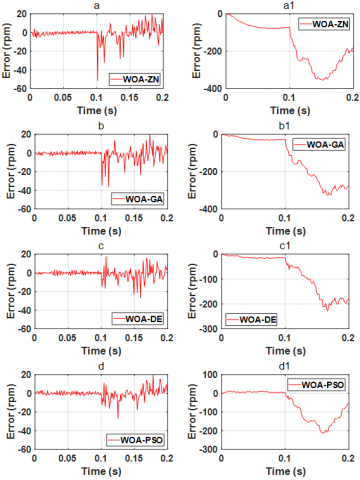Figure 4. Tracking performance for constant speed under simulation

## 5.pngFigure 5. Tracking performance for sinusoidal command speed under simulation

It can be seen from Figure 5: (i) the difference value between maximum and minimum of the Relative Error curves are 6.8 rpm, -1.1 rpm, 11.2 rpm and 4.7 rpm respectively. (ii) Among the Cumulative Error curve, only the WOA-ZN is positive during 0 s to 0.02 s; the range are -190.0 rpm, -153.5 rpm, -114.1 rpm and -213.7 rpm respectively. (iii) The final values are -104.7 rpm, 129.7 rpm, -85.49 rpm and -148.1 rpm respectively. The above data show that: (i) the maximum fluctuation range of each algorithm is similar; (ii) The restraining load of WOA is better than other methods; (iii) the sum of absolute error of WOA is minimum.

Characteristic values include the Mean Variation (MV), the IAE, the Standard Deviation (SD) and the Control Effect (CE). Among them, the MV is the error between BLDCM and the set speed in unit time; the IAE is the anti-interference ability of the motor; the SD is the discrete situation of the motor speed. IAE is the most concerned indicator. The smaller MV and SD are under the premise of the smallest IAE, the better the curve control effect will be. The CE satisfies Eq. (13).

$C E=0.6 *|I A E|+0.2 *|M V|+0.2 *|S D|$              (13)

The indexes of each curve after calculation are shown in Table 1.

Table 1. Performance indicators for BLDCM simulation

 Algorithm IAE MV SD CE Step speed command ZN 7.1656 -0.8092 1.4385 4.4252 GA 7.6322 -1.0826 1.4346 4.6497 DE 7.1457 -0.9414 1.3697 4.3731 PSO 6.5119 -1.4496 1.3264 3.8825 WOA 6.2691 -0.8890 1.2964 3.8429 Sinusoidal speed command ZN 5.3156 -0.1508 1.1243 3.3841 GA 5.4405 2.5787 1.1160 4.0032 DE 5.2197 2.2151 1.1106 3.7969 PSO 5.5325 2.4983 1.1475 4.0486 WOA 4.7922 0.5697 1.1272 3.2147

The information of the indicators for WOA can be obtained from Table 1: (i) The IAE and SD are better than other algorithms, and the MV is only worse than ZN under the constant speed. (ii) At sinusoidal speed; IAE is better than other algorithms; MV is only worse than ZN; SD is better than PSO and slightly inferior to ZN, GA and the DE. (iii) In terms of the overall control effect (CE), the speed setting constant is increased by 13.16% (ZN), 17.35% (GA), 12.12% (DE) and 1.02% (PSO), with an average increase of 10.89%; when the speed is set as sinusoidal speed, it is increased by 5.00% (ZN), 19.70% (GA), 15.33% (DE) and 20.60% (PSO) respectively, with an average increase of 15.16%. Through the above analysis, when the set speed is constant speed: (i) the steady state value of WOA is slightly less than the expected value; (ii) there is a small amplitude oscillation near the steady-state value; (3) the sum of absolute oscillation error is the smallest. When the set speed is sinusoidal speed: (i) the steady-state value of WOA is slightly higher than the expected value; (ii) there is a small amplitude oscillation near the steady-state value; (iii) the sum of absolute oscillation error is the smallest. Therefore, the overall control effect of WOA is the best.

4.2 Experiments of BLDCM

## 6.png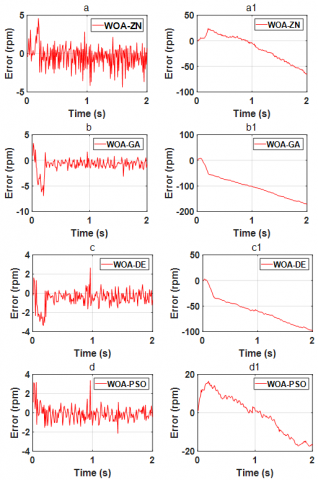Figure 6. Tracking performance for Constant command speed under experimental

## 7.png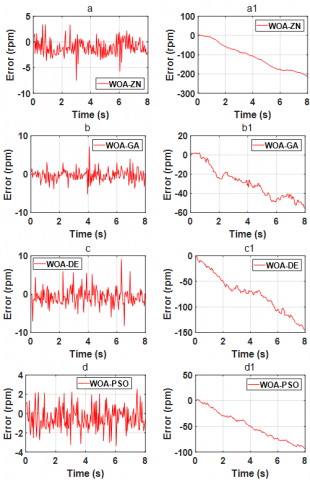Figure 7. Tracking performance for sinusoidal command speed under experimental

In the experiment of the BLDCM, the setting Constant speed was 300 rpm, and the setting Sinusoidal speed was 10 rad/s with the upper limit of 300 rpm and the lower limit of -300 rpm. The response curves of the error for the motor speed based on ZN, GA, DE, PSO and the WOA are shown in Figure 6 and Figure 7.

Experimental results are shown in Figure 6 and Figure 7. It can be seen from Figure 6 that: (i) the range of the Relative Error curves are 0.2 rpm, -3.6 rpm, -1.1 rpm and 1.2 rpm respectively. (ii) Among the Cumulative Error curve, the WOA-ZN and the WOA-PSO are positive during 0 s to 1 s; the range are -41.1 rpm, -162.0 rpm, -95.9 rpm and -0.8 rpm respectively. (iii) The final values are -65.17 rpm, -170.8 rpm, -98.53 rpm and -16.33 rpm respectively. It shows that: (i) the fluctuation range of WOA is larger than that of ZN and PSO, and smaller than that of GA and DE. (ii) the above data shows that the rising speed of WOA is only lower than that of Zn and PSO. (iii) the sum of absolute error of WOA is minimum.

It can see from Figure 7 that: (i) the range of the Relative Error curves are -4.1 rpm, 2.0 rpm, 0.8 rpm and -0.8 rpm respectively; (ii) among the Cumulative Error curve, the WOA-GA is positive during 0 s to 0.6 s; the range are -212.2 rpm, -53.0 rpm, -145.3 rpm and -91.5 rpm respectively; the final values are -214.6 rpm, -55.4 rpm, -145.3 rpm and -93.7 rpm respectively. The above data show that: (i) the maximum fluctuation amplitude of WOA is only less than GA and DE; (ii) the rising speed of WOA in the initial stage is only slightly less than GA, (iii) the overall control effect of WOA is the best.

Table 2. Performance indices for BLDCM

 Algorithm IAE MV SD CE Step speed command ZN 16.8061 -16.3392 3.7719 7.5702 GA 17.3376 -17.3085 3.7855 7.6980 DE 16.9764 -16.9381 3.7911 7.5564 PSO 16.5675 -16.5325 3.7574 7.3855 WOA 16.4837 -16.4579 3.7818 7.3550 Sinusoidal speed command ZN 3.8790 -0.6615 0.4405 2.2832 GA 3.0828 -0.3708 0.3982 1.8551 DE 3.5322 -0.5317 0.4157 2.0961 PSO 3.2747 -0.6303 0.4347 1.9257 WOA 2.8060 -0.4138 0.4137 1.6836

Compared with other algorithms, the following information of WOA can be obtained from Table 2: (i) when following constant speed: the IAE is better than other algorithms, MV is worse than ZN, SD is slightly worse than ZN and PSO. (ii) When following the sinusoidal speed: the IAE is better than other algorithms, MV and SD are only better than GA. (iii) The SD of different algorithms is similar. Through the above analysis, when the set speed is constant speed: (i) the steady state value of WOA is slightly less than the expected value; (ii) there is a small amplitude oscillation near the steady-state value; (3) the sum of absolute oscillation error is the smallest. When the set speed is sinusoidal speed: (i) the steady-state value of WOA is slightly less than the expected value; (ii) there is a small amplitude oscillation near the steady-state value; (iii) The sum of absolute oscillation error is the smallest. Therefore, the overall control effect of WOA is the best. This paper takes IAE as the main criterion, because the input signal of single-chip microcomputer is discrete signal, the positive selected speed of Brushless DC motor is a combination of several constant speeds. Compared with the constant speed simulation of Brushless DC motor we get the conclusion that the experiment is basically consistent with the simulation.

5. Conclusion

This paper proposes a parameter tuning method for the controller of BLDCM based on WOA. The two groups of simulations and experiments show that the method follows the Constant speed and Sinusoidal speed well. By comparing with the other methods, the CE of WOA increases by 2.84% (ZN), 4.46% (GA), 2.67% (DE), 0.41% (PSO), with an average increase of 2.56% under the Constant speed, and increases by 26.26% (ZN), 9.24% (GA), 19.68% (DE), 12.57% (PSO), with an average increase of 16.93% under the Sinusoidal rotation speed.

The experimental results show that the speed control of BLDCM is improved obviously by the proposed method, but further research is needed. (i) In the experiment of constant speed of BLDCM, there is no significant difference in the accuracy of each algorithm due to the inability to add a fixed load. (ii) In the sinusoidal speed experiment, the proposed algorithm improves the motor control accuracy greatly, but there is still a large space for improvement. (iii) The experiment has only been verified by one kind of Brushless DC motor, and the proposed method has not been studied the control effect of different kinds motor.

Experiments show that this method is more accurate than the traditional algorithm, and the control accuracy is improved obviously in the complex speed requirement. With the increasing requirement of Brushless DC motor, the control mode of BLDCM will be more complicated. Therefore, the self-tuning method to parameters proposed in this paper may be a promising parameter processing algorithm to improve the accuracy of complex motor control. Future work also needs to pay attention to the universality and interpretability of self-tuning method to parameters.

Acknowledgment

This work is supported by the National Natural Science Foundation of China (61650106) and Supported by Foundation of Guilin University of Technology (GLUTQD2013007).

References

 Madichetty, S., Pullaguram, D., Mishra, S. (2019). A standalone BLDC based solar air cooler with MPP tracking for improved efficiency. CSEE Journal of Power and Energy Systems, 5(1): 111-119. 10.17775/CSEEJPES.2018.00410

 Dubey, M., Sharma, S.K., Saxena, R. (2020). Solar power‐driven position sensorless control of permanent magnet brushless DC motor for refrigeration plant. International Transactions on Electrical Energy Systems, 30(7): e12408. https://doi.org/10.1002/2050-7038.12408

 Yao, Y., Liu, C., Lee, C.H. (2018). Quantitative comparisons of six-phase outer-rotor permanent-magnet brushless machines for electric vehicles. Energies, 11(8): 2141. https://doi.org/10.3390/en11082141

 Cao, F. (2020). PID controller optimized by genetic algorithm for direct-drive servo system. Neural Computing and Applications, 32(1): 23-30. https://doi.org/10.1007/s00521-018-3739-z

 Ma, C., Tian, S., Xiao, X., Jiang, Y. (2020). Fuzzy Neural Network PID–based constant deceleration compensation device for the brakes of mining hoists. Advances in Mechanical Engineering, 12(7): 1687814020937568. https://doi.org/10.1177/1687814020937568

 El-Wakeel, A.S., Ellissy, A.E.E.K.M., Abdel-hamed, A.M. (2015). A hybrid bacterial foraging-particle swarm optimization technique for optimal tuning of proportional-integral-derivative controller of a permanent magnet brushless DC motor. Electric Power Components and Systems, 43(3): 309-319. https://doi.org/10.1080/15325008.2014.981320

 Zhou, G., Li, J., Tang, Z., Luo, Q., Zhou, Y. (2020). An improved spotted hyena optimizer for PID parameters in an AVR system. Mathematical Biosciences and Engineering, 17(4): 3767-3783. https://doi.org/10.3934/mbe.2020211

 Jing, Z. (2020). Application of genetic fuzzy immune PID algorithm in cruise control for commercial vehicles. AIP Advances, 10(9): 095007.

 Cui, X., Chen, Z., Yin, F. (2018). Differential evolution and local search based monarch butterfly optimization algorithm with applications. International Journal of Computational Intelligence Systems, 12(1): 149-163. https://doi.org/10.2991/ijcis.2018.25905188

 Yu, Y., Xu, Y., Wang, F., Li, W., Mai, X., Wu, H. (2020). Adsorption control of a pipeline robot based on improved PSO algorithm. Complex & Intelligent Systems, pp. 1-7. https://doi.org/10.1007/s40747-020-00190-z.

 Feroz Mirza, A., Mansoor, M., Ling, Q., Khan, M.I., Aldossary, O.M. (2020). Advanced variable step size incremental conductance MPPT for a standalone PV system utilizing a GA-tuned PID controller. Energies, 13(16): 4153. https://doi.org/10.3390/en13164153

 Wang, Y., Wang, Q., Fu, H. (2020). Online high performance genetic algorithm based sliding mode control for controllable pitch propeller. Processes, 8(8): 953. https://doi.org/10.3390/pr8080953

 Chakkarapani, K., Thangavelu, T., Dharmalingam, K., Thandavarayan, P. (2019). Multiobjective design optimization and analysis of magnetic flux distribution for slotless permanent magnet brushless DC motor using evolutionary algorithms. Journal of Magnetism and Magnetic Materials, 476: 524-537. https://doi.org/10.1016/j.jmmm.2019.01.029

 Chao, C.T., Liu, M.T., Wang, C.J., Chiou, J.S. (2020). A fuzzy adaptive controller for cuckoo search algorithm in active suspension system. Journal of Low Frequency Noise, Vibration and Active Control, 39(3): 761-771. https://doi.org/10.1177/1461348418811473

 Keshavarzian, H., Daneshjou, K. (2020). PSO-based online estimation of aerodynamic ground effect in the backstepping controller of quadrotor. Journal of the Brazilian Society of Mechanical Sciences and Engineering, 42(11): 1-10. https://doi.org/10.1007/s40430-020-02614-w

 Huang, J.G., Hui, F., Jie, W. (2019). A PI controller optimized with modified differential evolution algorithm for speed control of BLDC motor. Automatika: časopis za automatiku, mjerenje, elektroniku, računarstvo i komunikacije, 60(2): 135-148. https://doi.org/10.1080/00051144.2019.1596014

 Zhu, D.C., Du, B.L., Zhu P.C., Chen, S.Y. (2020). Constant Force PID Control for Robotic Manipulator Based on Fuzzy Neural Network Algorithm. Complexity. https://doi.org/10.1155/2020/3491845

 Elçi, E.M., Grimm, J., Ding, L., Nasrawi, A., Garoni, T. M., Deng, Y. (2018). Lifted worm algorithm for the Ising model. Physical Review E, 97(4): 042126. https://doi.org/10.1103/PhysRevE.97.042126

 Tran, H.K., Chiou, J.S., Dang, V.H. (2020). New Fusion Algorithm-Reinforced Pilot Control for an Agricultural Tricopter UAV. Mathematics, 8(9): 1499. https://doi.org/10.3390/math8091499

 Napole, C., Barambones, O., Calvo, I., Velasco, J. (2020). Feedforward compensation analysis of piezoelectric actuators using artificial neural networks with conventional PID controller and single-neuron PID Based on Hebb learning rules. Energies, 13(15): 3929. https://doi.org/10.3390/en13153929

 Qin, J.H., Luo, J., Chuang, K.C., Lan, T.S., Zhang, L.P., Yi, H.A. (2020). Stable balance adjustment structure of the quadruped robot based on the bionic lateral swing posture. Mathematical Problems in Engineering. https://doi.org/10.1155/2020/1571439